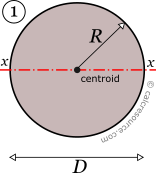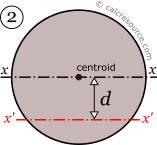## Moment of Inertia of a Circle

This tool calculates the moment of inertia I (second moment of area) of a circle. Enter the radius 'R' or the diameter 'D' below. The calculated result will have the same units as your input. Please use consistent units for any input.

 R = ...or D = The result is: Ix = Enter an axis offset: d = Ix' =## Definitions

The moment of inertia of circle with respect to any axis passing through its centroid, is given by the following expression:

$I = \frac{\pi R^4}{4}$

where R is the radius of the circle.

### Parallel Axes Theorem

The moment of inertia of any shape, in respect to an arbitrary, non centroidal axis, can be found if its moment of inertia in respect to a centroidal axis, parallel to the first one, is known. The so-calledParallel Axes Theorem is given by the following equation:

$I' = I + A d^2$

where I' is the moment of inertia in respect to an arbitrary axis, I the moment of inertia in respect to a centroidal axis, parallel to the first one, d the distance between the two parallel axes and A the area of the shape ($$=\pi R^2$$ in case of a circular area).

### Dimensions

The dimensions of moment of inertia (second moment of area) are $$[Length]^4$$.

### Mass moment of inertia

In Physics the term moment of inertia has a different meaning. It is related with the mass distribution of an object (or multiple objects) about an axis. This is different from the definition usually given in Engineering disciplines (also in this page) as a property of the area of a shape, commonly a cross-section, about the axis. The termsecond moment of area seems more accurate in this regard.

### Applications

The moment of inertia (second moment or area) is used in beam theory to describe the rigidity of a beam against flexure (see beam bending theory). The bending moment M applied to a cross-section is related with its moment of inertia with the following equation:

$M = E\times I \times \kappa$

where E is the Young's modulus, a property of the material, and κ the curvature of the beam due to the applied load. Beam curvature κ describes the extent of flexure in the beam and can be expressed in terms of beam deflection w(x) along longitudinal beam axis x, as: $$\kappa = \frac{d^2 w(x)}{dx^2}$$. Therefore, it can be seen from the former equation, that when a certain bending moment M is applied to a beam cross-section, the developed curvature is reversely proportional to the moment of inertia I. Integrating curvatures over beam length, the deflection, at some point along x-axis, should also be reversely proportional to I.